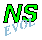## Lamb dipole crossing a ridge

In this simulation a Lamb dipole is moving through the domain in the positive x-direction. Along the y-axis there is a smooth cosine-shaped ridge, which the Lamb dipole will hit perpendicular. There is no background rotation present.

The computation is actually purely two-dimensional, but introducing a third dimension for illustrative purposes only, a "cross-section" of the ridge looks like this:Cross-section of a cosine-shaped ridge along the y-axis and uniform in the y-direction. The maximum height of the ridge (at x=0) is 2A, and its width is w. In the example shown here A=0.2 and w=1.

The dipole is initially placed to the left of the ridge: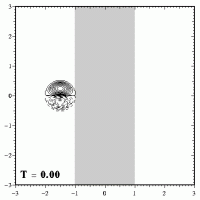Initial situation: a Lamb dipole of radius R=0.5 is placed to the left of the ridge. This ridge is the grey-shaded region, with its maximum (of 2A=0.4) at x=0. Positive vorticity contours are solid, negative vorticity contours are dashed.

The dipole will now move to the right because of its self-induced motion and start climbing the ridge. The general effect of a bottom topography is that when a dipole climbs a ridge it will weaken and widen, since (in the absence of viscosity):

```      vorticity
----------- = constant      (no background rotation)
fluid depth
```
Both dipole halves are affected in the same way by this topography effect (provided there is no background rotation, as is the case here): both halves become weaker as the fluid depth decreases. Consider for instance a dipole with two halve of strength 20 at fluid depth 1 (the default value):
```                      fluid depth = 1    fluid depth = 0.5

Constant A =  20 =    +20 / 1     =      +10 / 0.5
Constant B = -20 =    -20 / 1     =      -10 / 0.5
```
Both dipole halves have a strength of 10 at fluid depth 0.5.

This means that the dipole will not move away from the straight path it would follow if there was no topography. The weakening and widening of the dipole can be seen clearly in these plots: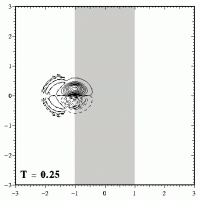While the dipole climbs the ridge (the grey shaded region), it weakens and wides, as can clearly be seen from the contours of vorticity drawn in these pictures. As the dipole moves, it leaves behind a tail of low-value vorticity.

When the dipole has crossed the maximum height of the ridge (which is at x=0), it descends from the ridge. During that descend the dipole will shrink again and become stronger, as shown here: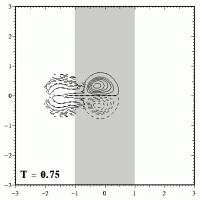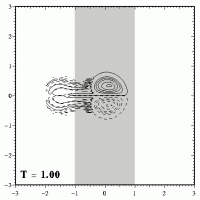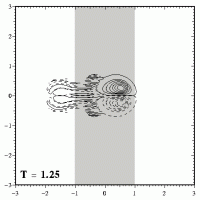At T=1 the dipole has just crossed the top of the ridge. While the dipole descends from the ridge (the grey shaded region), the dipole shrinks and becomes stronger.

Once the dipole has left the ridge, the dipole has the same size and strength again, which it had before it started climbing the ridge. After leaving the ridge, the dipole will therefore move further to the right, like an undisturbed Lamb dipole does.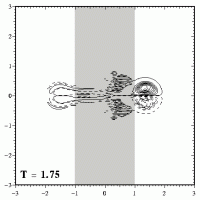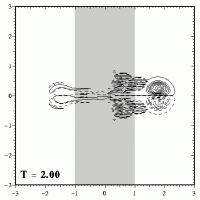The dipole has left the ridge and evolves further as an ordinary Lamb dipole.

The motion across the ridge can be seen nicely in an MPEG movie (700kb; 126 frames), with the evolution until T=2.5, when the dipole has reached the right boundary.

#### Other ridge heights

For lower positive values of A the disturbance of the ridge is of course less and for A=0 there is no disturbance at all: the dipole moves all the way as an ordinary Lamb dipole. For negative A the nature of the disturbance is the same but reversed: the dipole first becomes smaller and stronger as it descends into the trough, and it widens and weakens again when it climbs out of the trough.
For values of A larger than the A=0.2 used above the disturbance is bigger. For A=0.4 it appears that the dipole is torn apart, but such a high ridge is unacceptable: motion across its top -- where the height is 0.8 with respect to the default fluid depth of 1 -- can hardly be assumed to be still two-dimensional. Similarly, a trough which is too deep is unacceptable in these 2D model simulations.
```
```

## Lamb dipole crossing a ridge -- with background rotation

If there is a background rotation in the system, then the results can be different, depending on the strength of the rotation. A weak rotation may only deflect the dipole, whereas a strong rotation can reflect the dipole agains the ridge.